SYLLABUS  Previous: 2.3 Lax-Wendroff  Up: 2 FINITE DIFFERENCES  Next: 2.5 Implicit Crank-Nicholson

## 2.4 Leap-frog, staggered grids

Slide : [ Staggered leapfrog Scheme - Code - Run || VIDEO login]

The leap-frog algorithm is often used for the propagation of waves, where a low numerical damping is required with a relatively high accuracy. Relying on two functions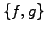to approximate the scalar wave equation (1.3.1#eq.2) in flux-conservative form, staggered grids (where the mesh points are shifted with respect to each other by half an interval, as in fig.2.4#fig.4) are used to evaluate centered differences with an accuracy in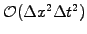: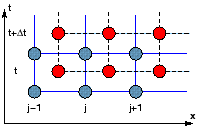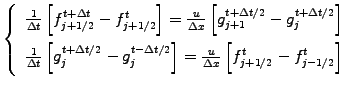(2.4.0#eq.9)

The construction of the leap-frog algorithm suggests how the Maxwell equations can be discretized using finite differences in the time domain (FDTD) to solve vector equations in terms of the electric and the magnetic fields evaluated on staggered grids (exercise 2.08). The leap-frog algorithm has been implemented in JBONE as
      for (int j=1; j<=n; j++) {                  //1st equation
fp[j]=f[j] -beta*(g[j]-g[j-1]); }
fp=f -beta*(g-g[n]);
for (int j=0; j<=n-1; j++) {                //2nd equation
gp[j]=g[j] -beta*(fp[j+1]-fp[j]); }
gp[n]=g[n] -beta*(fp-fp[n]);

Special care is required when starting the integration, since the initial condition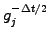determines the direction of propagation (exercise 2.04). In addition to the periodic boundaries shown here above, Dirichlet and absorbing boundary conditions are also frequently used (exercises 2.04 and 2.08).

 Numerical experiments: leap-frog Examine the propagation and the damping of harmonic functions; try to assess the numerical properties directly from your observations. Study the initialization with PhysDataValue in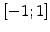.

By substitution, note that the leap-frog scheme is equivalent to the implicit 3 levels scheme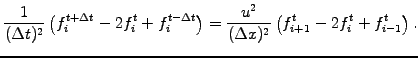(2.4.0#eq.10)

where the linear problem has been solved explicitly in an elegant manner.

SYLLABUS  Previous: 2.3 Lax-Wendroff  Up: 2 FINITE DIFFERENCES  Next: 2.5 Implicit Crank-Nicholson